# Bernstein-Rogosinski summation method

One of the methods for summing Fourier series; denoted by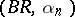. A trigonometric series(*)

is summable by the Bernstein–Rogosinski method at a point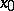to the valueif the following condition is satisfied: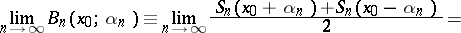where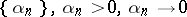, is a sequence of numbers, and where the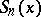are the partial sums of the series (*).

W. Rogosinski  first (1924) considered the case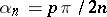, whereis an odd number, and then (1925) the general case. S.N. Bernstein [S.N. Bernshtein]  considered (1930) the case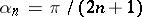. The-method sums the Fourier series of a function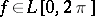in the casesandat the points of continuity of the function to its value and is one of the regular summation methods.

The Bernstein–Rogosinski sumsare employed as an approximation procedure. In both cases described above they realize an approximation of the same order as the best approximation for functions of the classesand.